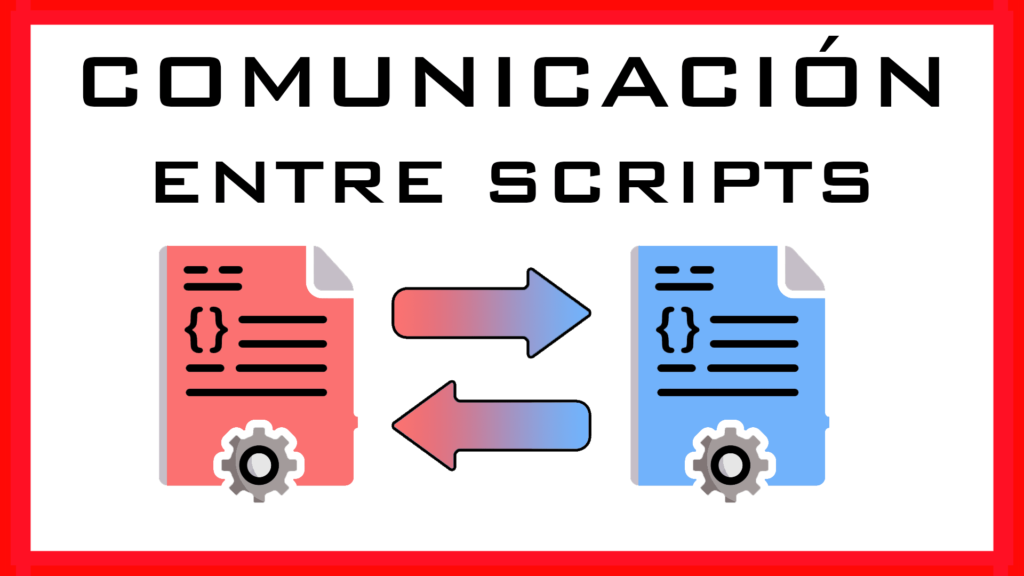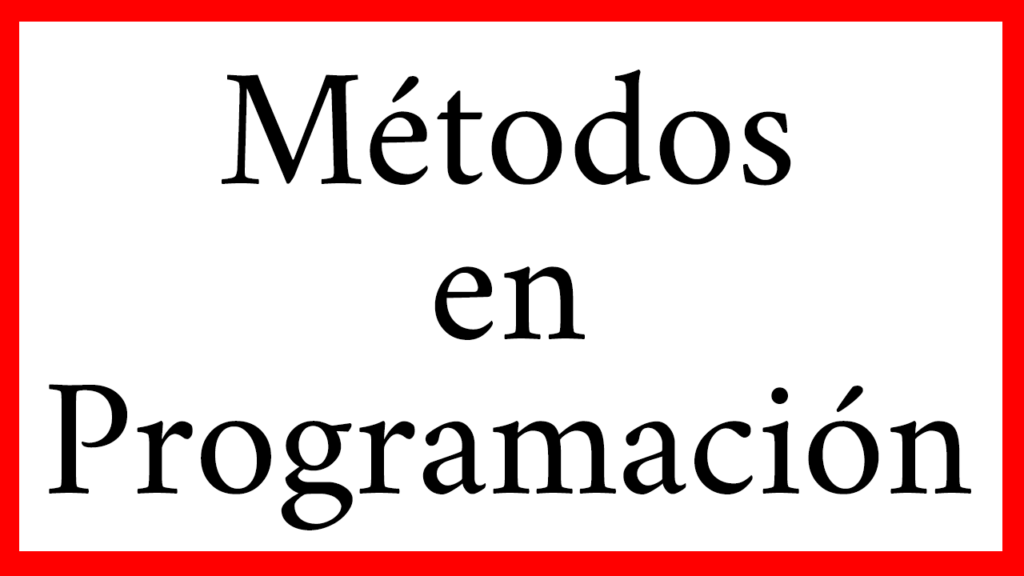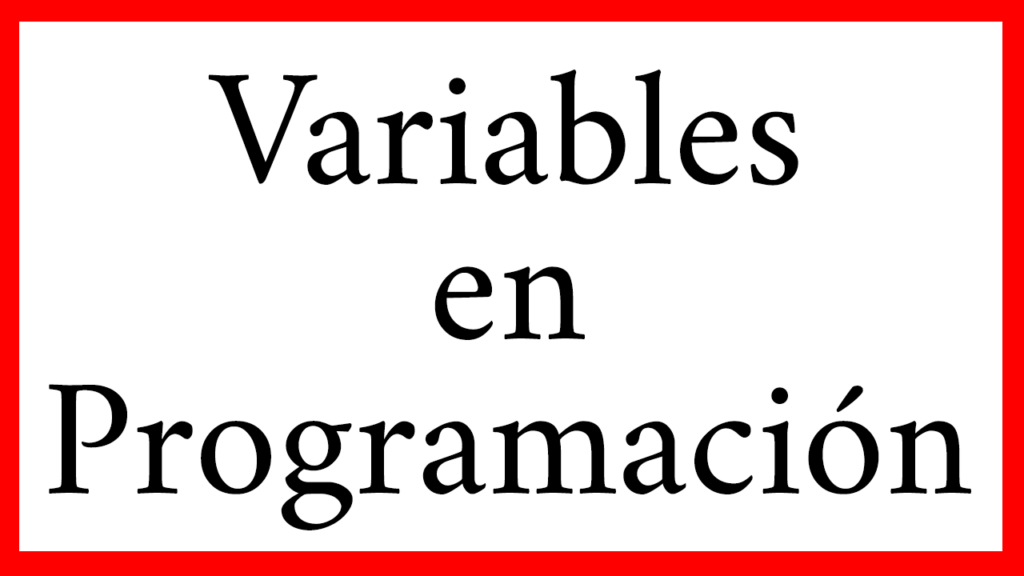# Basic Programming## IF Statement – Decision Control Structure

Introduction The IF statement allows us to make a decision based on whether a condition is true or false. The simple decision is to perform an action if the condition is true and otherwise do nothing. The double decision allows us to perform a second action if the condition is false. In the following video …

## How to read VARIABLES from other Scripts. Example in C# Unity

Introduction In this article we will see how to read variables that are defined in a script from a second script. In the following video you can see how to use the variables of other scripts step by step. Procedure for using variables from other scripts 1. Let 2 scripts A and B. In A …

## How to call FUNCTIONS defined in another Script. Examples in C# Unity

Introduction In this article we will see how to execute functions defined in one script from a second script. First we look at the generic procedure. Procedure 1. Let 2 Scripts A and B. In Script A is the function we want to call. In Script B we are going to call the function that …

## Modularization in Programming

Introduction In this article we will see what MODULARIZATION is in programming and why it is important to apply it. We will also see examples of applications in C# language oriented to Unity. What is MODULARIZATION in Programming and what is it for? The word modularization comes from module, whose textual definition is: ” each …

Introduction In this article we will look at what a programming paradigm is for information purposes and give some examples. The first generations of computers were programmed using machine language, i.e. a sequence of instructions was given that the machine understood. As it was difficult to remember the codes of these instructions, the Assembler language …## Communication between Scripts – Examples in Unity

Introduction In this article we are going to see how to use functions that are defined in a Script from any other Script. Communication between Scripts is an informal name for this problem, it means that being in one Script we will be able to access the context of another Script. This is important, especially …## Methods in Programming

Introduction In this article we see what is a method in programming, what they are for and how to declare them in C# language with concrete examples in a game project in Unity. The following video summarizes some of the information in this article. Activate English subtitles ¿What is a Method in Programming? In this …## Variables in Programming

Introduction In this article we are going to explain what is a variable in programming, what types of variables there are, what they are used for and show some examples of variables in C#, in the development of video games with Unity. The following video summarizes some of the information in this article. You can …